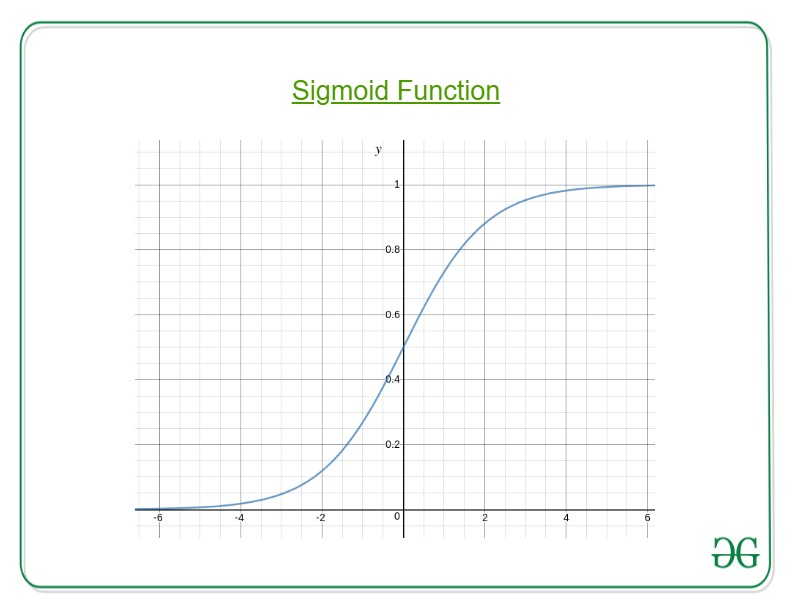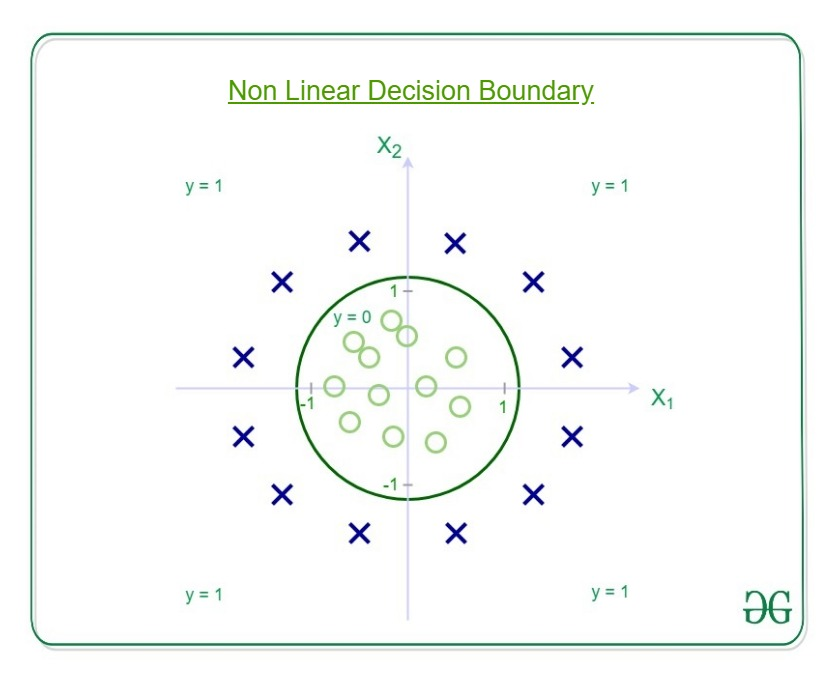# ML | Why Logistic Regression in Classification ?

Using Linear Regression, all predictions >= 0.5 can be considered as 1 and rest all < 0.5 can be considered as 0. But then the question arises why classification can’t be performed using it?

Problem –

Suppose we are classifying a mail as spam or not spam and our output is y, it can be 0(spam) or 1(not spam). In case of Linear Regression, hθ(x) can be > 1 or < 0. Although our prediction should be in between 0 and 1, the model will predict value out of the range i.e. maybe > 1 or < 0.

So, that’s why for a Classification task, Logistic/Sigmoid Regression plays its role.Here, we plug θTx into logistic function where θ are the weights/parameters and x is the input and hθ(x) is the hypothesis function. g() is the sigmoid function.It means that y = 1 probability when x is parameterized to θ

To get the discrete values 0 or 1 for classification, discrete boundaries are defined. The hypothesis function cab be translated asDecision Boundary is the line that distinguishes the area where y=0 and where y=1. These decision boundaries result from the hypothesis function under consideration.

Understanding Decision Boundary with an example –
Let our hypothesis function beThen the decision boundary looks likeLet out weights or parameters be –So, it predicts y = 1 ifAnd that is the equation of a circle with radius = 1 and origin as the center. This is the Decision Boundary for our defined hypothesis.

My Personal Notes arrow_drop_upIf you like GeeksforGeeks and would like to contribute, you can also write an article using contribute.geeksforgeeks.org or mail your article to contribute@geeksforgeeks.org. See your article appearing on the GeeksforGeeks main page and help other Geeks.

Please Improve this article if you find anything incorrect by clicking on the "Improve Article" button below.

Article Tags :
Practice Tags :

Be the First to upvote.

Please write to us at contribute@geeksforgeeks.org to report any issue with the above content.